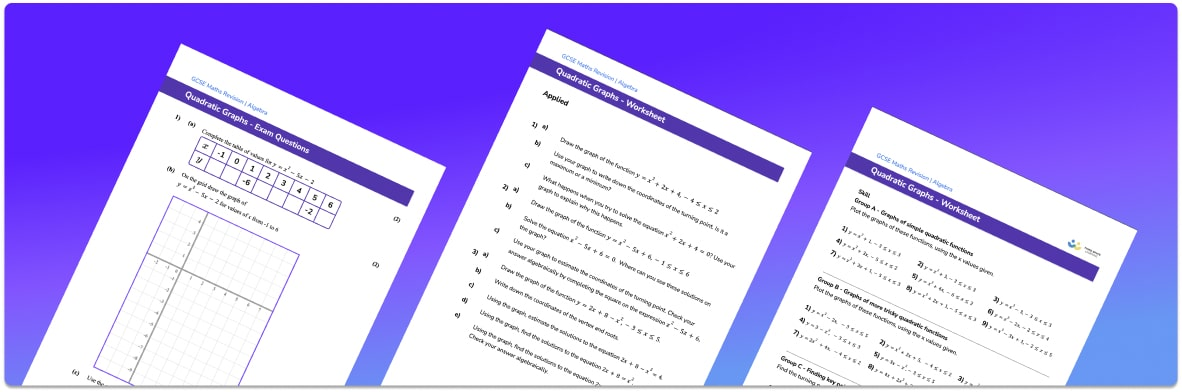• Section 1 of the quadratic graphs worksheet contains 20+ skills-based quadratic graphs questions, in 3 groups to support differentiation
• Section 2 contains 3 applied quadratic graphs questions with a mix of worded problems and deeper problem solving style questions
• Section 3 contains 3 foundation and higher level GCSE quadratic graphs exam questions
• Answers and a mark scheme for all quadratic graphs questions are provided
• Questions follow variation theory with plenty of opportunities for students to work independently at their own level
• All questions created by fully qualified expert secondary maths teachers
• Suitable for GCSE maths revision for AQA, OCR and Edexcel exam boards

• This field is for validation purposes and should be left unchanged.

You can unsubscribe at any time (each email we send will contain an easy way to unsubscribe). To find out more about how we use your data, see our privacy policy.

### Quadratic graphs at a glance

Quadratic graphs are a way of visually representing quadratic functions. Quadratic functions are functions in which the highest power of the variable is 2 and may also contain terms in x or constants. The general form for a quadratic function is y=ax2+bx+c.

There are two main methods for plotting graphs of quadratic functions. The first is to use the equation of the line to create a table of values of x and y. These can then be plotted as coordinates on a set of axes.

The second method for plotting quadratics is to create a sketch of the graph. When sketching quadratics, we need to determine the y intercept of the graph, the x intercepts of the graph and the turning point, or vertex, of the graph. If the equation of the line is y=ax2+bx+c, then the y intercept is c and the x intercepts are the roots of the equation ax2+bx+c=0 (since y is 0 along the x axis). The roots to this quadratic equation could be found by factorising or using the quadratic formula. The turning point of the graph can be found by completing the square.

A quadratic function will always produce either a u or n shaped graph called a parabola. If the coefficient of x2 is positive, it will be u shaped and if it is negative then it will be n shaped. Quadratics must be drawn as a smooth curve.

Looking forward, students can then progress to additional types of graph worksheets and on to more algebra worksheets, for example a factorising worksheet or simultaneous equations worksheet.For more teaching and learning support on Algebra our GCSE maths lessons provide step by step support for all GCSE maths concepts.

## Do you have KS4 students who need more focused attention to succeed at GCSE?There will be students in your class who require individual attention to help them succeed in their maths GCSEs. In a class of 30, it’s not always easy to provide.

Help your students feel confident with exam-style questions and the strategies they’ll need to answer them correctly with our dedicated GCSE maths revision programme.

Lessons are selected to provide support where each student needs it most, and specially-trained GCSE maths tutors adapt the pitch and pace of each lesson. This ensures a personalised revision programme that raises grades and boosts confidence.

Find out more